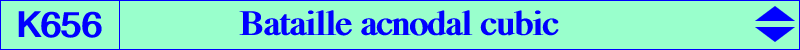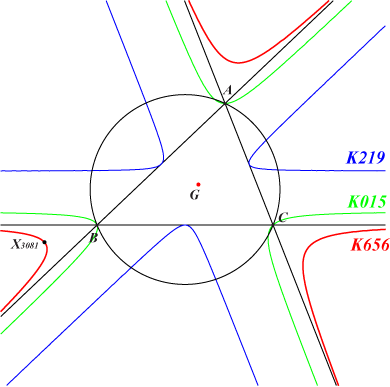(x + y + z)^3 = 27 x y zX(2), X(3081), X(6545), X(8027), X(8028), X(8029), X(8030), X(8031), X(8032), X(23610), X(23611), X(23612), X(23613), X(23614), X(23615), X(23616) points at infinity of ABC sidelines (inflexion points) barycentric cube of any point on the line at infinity : X(3081) is that of X(30)K656 is an acnodal cubic with singular point the centroid G of ABC. It has three real inflexional asymptotes which are the sidelines of ABC. It is related to K015 in several ways. It is mentioned in a paper by Michel Bataille : On the Foci of Circumparabolas, Forum Geometricorum Volume 11 (2011) 57–63. Locus properties : K656 is the envelope of the trilinear polars of points on the inscribed Steiner ellipse. See the related cubic K244 and also Generalization 1 below. K656 is the barycentric cube of the line at infinity. Recall that the Steiner inellipse is the barycentric square. See Generalization 2 below. The trilinear cube of the line at infinity is K244, hence K656 is the barycentric product X(6) × K244. Two parabolas, one inscribed Pi and one circumscribed Pc, with the same infinite point meet at two other isotomic conjugate points M1, M2 lying on K015. These parabolas are homothetic and the center of homothety P lies on K656 (Michel Bataille). Recall that h(P, 4) maps Pc onto Pi. The centroid of the tangential triangle of a circumscribed parabola lies on K656 since it is the center of homothety above (Michel Bataille). The arithmetic and geometric means of the barycentric coordinates of a point are equal if and only if this point lies on K656. When "geometric" is replaced by "harmonic" we find the cubic K015. See here for other related properties (Angel Montesdeoca, 2019-07-13). Other properties : K656 is the homothetic of K015 under h(G, 3/2) and also the anticomplement of K700. The Hessian of K656 is K219, the complement of K015. More generally, the nth Hessian of K656 is the homothetic of K015 under the homothety with center G, ratio 3/2 (-1/3)n. It follows that two cubics of the group K015, K219, K656, K700 are homothetic at G. More generally, the homothety h(G,T) maps K656 to a cubic K(T) of the same type with equivalent equations : (y+z-2 x) (x-2 y+z) (x+y-2 z) + 3 T (x+y+z) (x^2-x y+y^2-x z-y z+z^2) = 0 (-2 x+y+z-T (x+y+z)) (x-2 y+z-T (x+y+z)) (x+y-2 z-T (x+y+z)) + T^3 (x+y+z)^3 = 0 With T = 2/3, -1/3, 1, -1/2 respectively, we find the four cubics above. With T = 1/3, we find K1310. K(T) and K(-T) are symmetric about G. See K219 and K1310 for example. The hessian of K(T) is K(-T/3). See K656 and K219 for example.Generalization 1 and construction : Let C(P) be the inscribed conic with perspector P = p:q:r and L(P) the trilinear polar of P. The envelope of the trilinear polar L(M) of point M on C(P) is the unicursal cubic K(P) with node P and equation : (qrx+rpy+pqz)^3 - 27 p^2q^2r^2 xyz = 0. If N is the trilinear pole of the tangent at M to C(P), the point X = PN /\ L(M) lies on K(P) and it is the point of tangency of L(M) with K(P). Note that N lies on L(P). K(P) meets the sidelines of ABC at three points U, V, W which also lie on L(P). These points are three real inflexion points on the cubic and the inflexional tangents are the sidelines of ABC. K(P) is acnodal for any finite point P. In particular, K(X2) = K656, K(X76) = K244.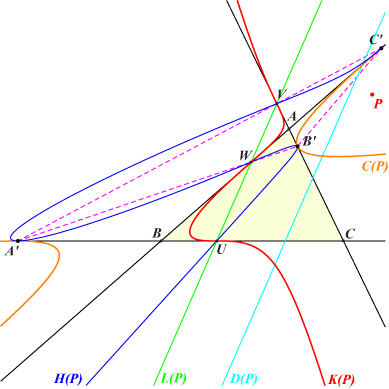The Hessian H(P) of K(P) is also an acnodal cubic with node P obviously passing through the points U, V, W. H(P) is the image of K(P) under the homology with pole P, axis L(P) which transforms the line at infinity into the line D(P), the image of L(P) under the homothety h(P, 1/4). This homology becomes the homothety h(G, -1/3) when P = G. This homology is given by the matrix :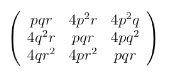With I = 1+t : t : -1-2t on the line at infinity, the points mentioned above are : N = P x I (barycentric product) on L(P), M = P x I^2 on C(P), X = P x I^3 on K(P), S = (1+3t)^2 : (2+3t)^2 :1, antipode of I^2 on the Steiner inellipse, T = S x N on H(P) J = 1+3t : -2-3t : 1, infinite point of the polar line of I in the Steiner inellipse, T' = M x J on H(P), center of the polar conic of T in K(P). T and T' are corresponding points on H(P). The envelope of TT' is the Cayleyian which splits into C(P) and the polar conic of P in K(P).Generalization 2 (Angel Montesdeoca) : Let U = u :v : w be a point with isotomic conjugate tU = 1/u : 1/v : 1/w. Consider the mapping F which transforms U and tU into the barycentric product of their respective complements cU and ctU. Hence F is given by V = F(U) = F(tU) = u (v + w)^2 : v (w + u)^2 : w (u + v)^2. Now, let C(P) be the circum-conic with perspector P = p : q : r and equation p y z + q z x + r x y = 0 whose isotomic transform is the line L(P) with equation p x + q y + r z = 0, clearly the trilinear polar of tP. F transforms C(P) and L(P) into a nodal cubic K(P) with node N which is the crossconjugate of P^2 and P. N = p / (- p + q + r) : q / (p - q + r) : r / (p + q - r) = P x taP, where aP is the anticomplement of P. K(P) meets the sidelines of ABC at the traces of the trilinear polars of P and (taP)^2, the tangents at these latter points being the sidelines of ABC. Note that these trilinear polars are parallel. K(P) has only three inflexion points and one is always real. These points lie on the line ∆(L) with equation : ∑ (- p + q + r) [ p (- p + q + r) + 2 q r ] x = 0. When P = X(2), the cubic K(P) is K656 and L(P), C(P) are the line at infinity, the Steiner ellipse respectively. ∆(L) is also the line at infinity.K656 and the Stammler parabolas The Stammler circles are the three circles (apart from the circumcircle), that intercept the sidelines of a reference triangle in chords of lengths equal to the corresponding side lengths a, b, c. The centers of these circles form an equilateral triangle known as the Stammler triangle. See here. In a similar way, a Stammler parabola (P) meets the sidelines BC, CA, AB at U and U', V and V', W and W' such that UU' = a, VV' = b, WW' = c. This was first introduced in the thread Paraboles de Stammler (2018-10-18, in French) where proofs and details are given. In particular, it is proven there that, for a given U on BC, there is one and only one (possibly decomposed) such parabola. When U traverses the line BC, the envelope of (P) is the cubic K656.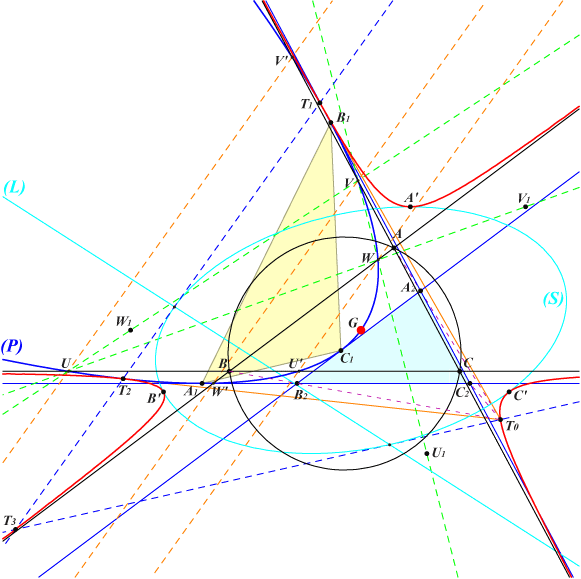Comments and constructions (most of them from the thread given above and others obtained from properties of K015) U1 = –2 : 1 : 4, V1 = 4 : –2 : 1, W1 = 1 : 4 : –2. In vectors, we have CU1 = AG, AV1 = BG, BW1 = CG and UU' = BC, VV' = CA, WW' = AB. The lines UV', VW', WU' are parallel. When U is given on BC, U' is entirely defined and V = AC /\ UW1 giving V', W = AB /\ UV1 giving W' hence (P) is defined. T1, T2 are the contacts of (P) and K656. The line T1T2 is tangent to (S), the homothetic of the Steiner ellipse under h(G, 3/2). (S) is tritangent to K656 at A', B', C', the images of A, B, C under the same homothety. This line T1T2 meets the cubic again at T3. The tangents at T1, T2 to (P) and K656 meet at T0 on the cubic and the line T0T3 is also tangent to (S). (L) is the polar of T3 in (S). It is parallel to the axis of (P). The homothethy h(T0, 3/4) maps the triangle ABC onto A2B2C2 and (P) is inscribed in A2B2C2 where A1, B1, C1 are the points of tangency.